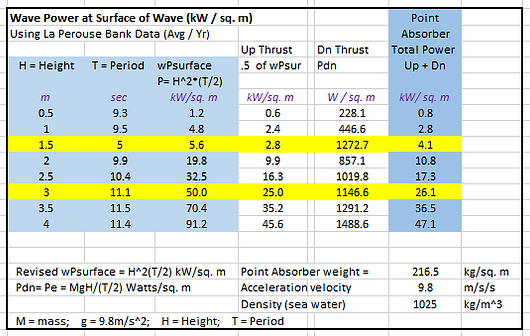Wave Power Density & Energy Conversion Efficiency     Assumptions

Wave Power Density

The accuracy of the formulas and equations for calculating the power density of wave energy is not very accurate at this time1 (3rd quarter 2015) specifically for determining the wave power density per sq. m acting vertically on a point absorber.

Consequently for the purposes of this calculation example a "best guess" formula is used which results in a power density estimate of 4.1 kW per sq. m for the up and down thrust on the 4 m diameter, 2,500 kg point absorber of Neptune 3 from a 1.5 m High wave with a 5 second Period. See calculation worksheet below.

In the near future, we expect to have accurate emperical data for this particular situation as a result of our testing of waves in the Georgia Strait which we will be comparing with the estimate used here.

Wave Energy Conversion Efficiency

This is also a relatively unknown quantity that is publicly available at this time.

With respect to the direct drive alternator power take off (PTO) used in Neptune 3, it is estimated that  point absorber WEC devices have an average efficiency of converting available wave power to electricity of 70% efficiency.

For the purposes of this calculator example, we are assuming  a 60% Efficiency for Wave Energy Conversion.

1.  Influence of Wave Resource Assessment Methods on Wave Power Production Estimates,
B Robertson, et al  Mechanical Engineering, University of Victoria, Canada, 2015

Click X  to close this note:

Stephen Salter's 1974 invention became known as Salter's duck or nodding duck, although it was officially referred to as the Edinburgh Duck.

In small scale controlled tests, the Duck's curved cam-like body can stop 90% of wave motion and can convert 90% of that to electricity
giving 81% efficiency.

https://en.wikipedia.org/wiki/Wave_power

Each Pelamis system is rated at approximately 750kW and the mechanical to electrical conversion efficiency is quoted to be 70 – 80%
(St Germain, Univ. of Victoria Masters Thesis, Mechanical Enginering, 2005)

Laurent Marquis CEO of Wave Star A/S (Denmark)

in a poster paper presented at ICOE 2014
reported that they would achieve wave energy conversion efficiency of 73%

by 2019, up from 65% in 2014.

NOTE:

For the purposes of this calculator example, we are assuming

a 60% Efficiency for Wave Energy Conversion.

more on WEC Efficiency

more on WEC Power Density

Another way to look wave energy density and efficiency is to start with the raw wave power determine an efficiency percentage that matches actual output.

Several formulas have been proposed for calculating wave power based on the formula: P = TH^2  such as:  .5H^2T  or .57H^2T or .59H^2T
(where T is the wave period and H is the wave height)

https://en.wikipedia.org/wiki/Wave_power

There are problems with using formulas based on "H^2T" here because they result in units of kW per lineal m of wave front and we are looking for units of power per sq. m.

A new more accurate formula will emerge in the future with integrals of periodic and random functions to integrate the essential cubic nature of waves and their variable period (speed) intermittant energy flow.

This formula we are using   P = H^2 * (T/2)
with modifications for up and down thrusts, yields the following:The results of this calculation are astounding -- consider:

Raw Energy Density / Sq. m    X   Efficiency  =  Electricity

Peak Power Solar   =      1.0  kW / sq. m       20%  =   .2 kW / sq. m

30 MPH Wind
*      =      3.2  kW / sq m        25%  =   .8 kW / sq. m

Average Tidal         =    3.0  kW / sq. m       30%  =   .75 kW / sq. m

Bay of Fundy Tidal =    18   kW / sq. m       30%  =   5.4 kW / sq. m

1.5 m Wave * *       =    4.1  kW / sq. m       50%  =   2.0 kW / sq. m

3.0 m Wave           =    26   kW / sq. m       50%  =  13.0 kW / sq. m

*  30 MPH wind = 13.4 m/s (at .625*A*V^3) = 1.5 kW / sq. m

multiplied by the 2.15 Iowa Energy Factor = 3.2 kW / sq. m

http://www.iowaenergycenter.org/wind-energy-manual/wind-and-wind-power/wind-speed-and-power/

*

A giant assumption has been made here,

that the wave power calculated as per lineal m per wave front some how equates to power per sq. m.

2010+     2015           2016+        Comparisons